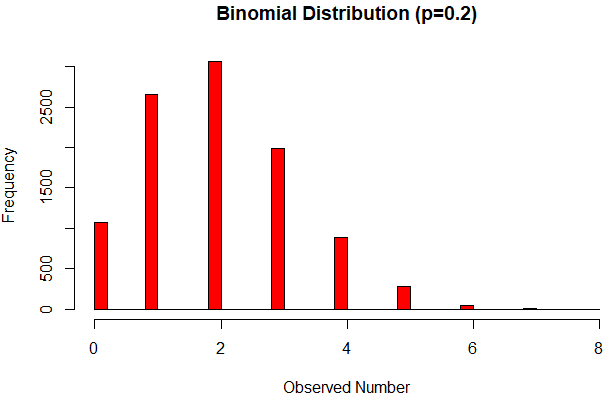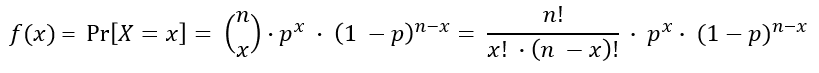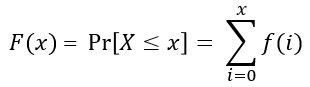# Binomial Distribution Calculator

Use this binomial probability calculator to easily calculate binomial cumulative distribution function and probability mass given the probability on a single trial, the number of trials and events. It can calculate the probability of success if the outcome is a binomial random variable, for example if flipping a coin. The calculator can also solve for the number of trials required.

Share calculator:

Embed this tool:
get code

## Using the Binomial Probability Calculator

You can use this tool to solve either for the exact probability of observing exactly x events in n trials, or the cumulative probability of observing X ≤ x, or the cumulative probabilities of observing X < x or X ≥ x or X > x. Simply enter the probability of observing an event (outcome of interest, success) on a single trial (e.g. as 0.5 or 1/2, 1/6 and so on), the number of trials and the number of events you want the probability calculated for. As long as the procedure generating the event conforms to the random variable model under a Binomial distribution the calculator applies. In other words, X must be a random variable generated by a process which results in Binomially-distributed, Independent and Identically Distributed outcomes (BiIID). For example, you can compute the probability of observing exactly 5 heads from 10 coin tosses of a fair coin (24.61%), of rolling more than 2 sixes in a series of 20 dice rolls (67.13%) and so on. See more examples below.

Under the same conditions you can use the binomial probability distribution calculator above to compute the number of attempts you would need to see x or more outcomes of interest (successes, events). For example, if you know you have a 1% chance (1 in 100) to get a prize on each draw of a lottery, you can compute how many draws you need to participate in to be 99.99% certain you win at least 1 prize (917 draws). Note that this example doesn't apply if you are buying tickets for a single lottery draw (the events are not independent).

Sequences of Bernoulli trials: trials in which the outcome is either 1 or 0 with the same probability on each trial result in and are modelled as binomial distribution so any such problem is one which can be solved using the above tool: it essentially doubles as a coin flip calculator.

## What is a Binomial Distribution?

The binomial distribution X~Bin(n,p) is a probability distribution which results from the number of events in a sequence of n independent experiments with a binary / Boolean outcome: true or false, yes or no, event or no event, success or failure. These are also known as Bernoulli trials and thus a Binomial distribution is the result of a sequence of Bernoulli trials. The parameters which describe it are n - number of independent experiments and p the probability of an event of interest in a single experiment. It is often used as a teaching device and the practical applications of probability theory and statistics due its many desirable properties such as a known standard deviation and easy to compute cumulative distribution function and inverse function.

The binomial probability distribution can be used to model the number of events in a sample of size n drawn with replacement from a population of size N, e.g. coin tosses, dice rolls, and so on. If the sampling is carried out without replacement they are no longer independent and the result is a hypergeometric distribution, although the binomial remains a decent approximation if N >> n.The above is a randomly generated binomial distribution from 10,000 simulated binomial experiments, each with 10 Bernoulli trials with probability of observing an event of 0.2 (20%).

## What is a Binomial Probability?

A probability for a certain outcome from a binomial distribution is what is usually referred to as a "binomial probability". It can be calculated using the formula for the binomial probability distribution function (PDF), a.k.a. probability mass function (PMF): f(x), as follows:where X is a random variable, x is a particular outcome, n and p are the number of trials and the probability of an event (success) on each trial. The term (n over x) is read "n choose x" and is the binomial coefficient: the number of ways we can choose x unordered combinations from a set of n. As you can see this is simply the number of possible combinations. In some formulations you can see (1-p) replaced by q.

Note that the above equation is for the probability of observing exactly the specified outcome. However, often when searching for a binomial probability formula calculator people are actually looking to calculate the cumulative probability of a binomially-distributed random variable: the probability of observing x or less than x events (successes, outcomes of interest).

## Binomial Cumulative Distribution Function (CDF)

The cumulative distribution function (CDF) of the Binomial distribution is what is needed when you need to compute the probability of observing less than or more than a certain number of events/outcomes/successes from a number of trials. The Binomial CDF formula is simple:Therefore, the cumulative binomial probability is simply the sum of the probabilities for all events from 0 to x. Our binomial distribution calculator uses the formula above to calculate the cumulative probability of events less than or equal to x, less than x, greater than or equal to x and greater than x for you. These are all cumulative binomial probabilities.

The inverse function is required when computing the number of trials required to observe a certain number of events, or more, with a certain probability. For this we use the inverse normal distribution function which provides a good enough approximation.

## Examples

Example 1: Coin flipping. If a fair coin (p = 1/2 = 0.5) is tossed 100 times, what is the probability of observing exactly 50 heads? What is the probability of observing more than 50 heads? Entering 0.5 or 1/2 in the calculator and 100 for the number of trials and 50 for "Number of events" we get that the chance of seeing exactly 50 heads is just under 8% while the probability of observing more than 50 is a whopping 46%. While in an infinite number of coin flips a fair coin will tend to come up heads exactly 50% of the time, in any small number of flips it is highly unlikely to observe exactly 50% heads.

Example 2: Dice rolling. If a fair dice is thrown 10 times, what is the probability of throwing at least one six? We know that a dice has six sides so the probability of success in a single throw is 1/6. Thus, using n=10 and x=1 we can compute using the Binomial CDF that the chance of throwing at least one six (X ≥ 1) is 0.8385 or 83.85 percent.

#### Cite this calculator & page

If you'd like to cite this online calculator resource and information as provided on the page, you can use the following citation:
Georgiev G.Z., "Binomial Distribution Calculator", [online] Available at: https://www.gigacalculator.com/calculators/binomial-probability-calculator.php URL [Accessed Date: 28 Sep, 2023].